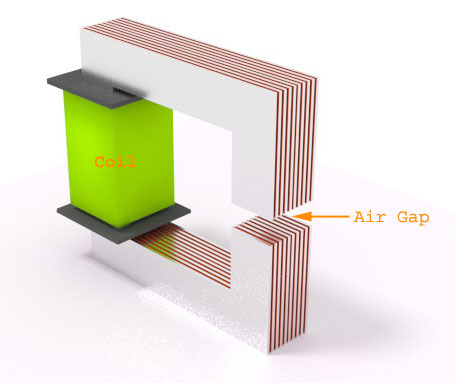# Magnetic Circuit with Air Gap

## Magnetic Circuit

When a magnetic flux is circulated or follow through a closed area or path, is called the magnetic circuit or when a magnetic field circulates in a closed path represented as lines of magnetic flux in a confined area is called Magnetic Circuit. This magnetic circuit forms with permanent magnets or electromagnets and confined to the path by magnetic cores consisting of ferromagnetic materials like iron etc.

## Magneto Motive Force (MMF)

A circulating force called Magneto Motive Force (MMF) or magnetic potential is responsible for establishing magnetic flux in a magnetic circuit. The MMF is equivalent to a number of wire carrying an electric current and has units of ampere turns.

MMF is the property of certain substances or phenomena that gives rise to a magnetic field and is analogous to electromotive force or voltage of electricity. If the flux is so divided that is enclosed to a portion of the device and part to another, the magnetic circuit is called parallel magnetic circuit and if all the flux is confined to a single closed loop, as in a ring-shaped electromagnet, the circuit is called a series magnetic circuit.

## Air Gap in Magnetic Circuit

Now if questioned what air gap in magnetic circuit is?, then the answer would be a prevention to the saturation in general. Air is a kind of insulator to electricity as well as magnetism, i.e. area with negativity is used for positive results. Like air, it can be as paint, gas, vacuum, aluminium etc. to prevent core saturation depending upon application of use.

But sometimes in transformer the air gap fails to prevent saturation caused by excessive AC voltage polarization.Air is a non-magnetic part of a magnetic circuit that connects serially and magnetically all the other parts in the circuit to make the flux to flow through the gap. Air gap has a significant character to enhance electrified parts to move physically in magnetic fields, without touching each other.
The air gap in magnetic circuit means the magnetic resistance, i.e. reluctance to the magnetic flux density. The reluctance of a magnetic circuit is proportional to its length and inversely proportional to its cross-sectional area and a magnetic property of the given material called its permeability. To calculate reluctance:

Magnetic Reluctance (R) = L/Aμμo
L = Length of circuit
A = Cross-sectional area of the circuit
μ = Permeability
μo = Relative magnetic permeability
The air gap is mostly used in applications where the magnetic saturation concludes as a high risk as magnetic saturation causes loss of inductance, increasing of current, power loss in the circuit. But implementation of air gap in a magnetic circuit influences the parameters of magnetic inductor also i.e. addition of reluctance (air) in the circuit changes the B-H curve (allows driving the inductor at higher current, therefore higher magnetic field strength, thus extending the range before magnetic saturation occurs), decrease the inductance and increasing the saturation current of magnetic inductor. The problem which an air gap solves in a core is the excessive flux produced with a high level of current in the windings.

Another phenomenon of flux in magnetic circuit is that most of the flux is confined to the intended path use of magnetic cores (ferromagnetic material), but a small amount of flux always complete its path through the surrounding air called the leakage flux. So whenever an air gap is put-up in magnetic core, flux fringes out into the neighboring air path and such paths for flux called flux fringing resulting in non-uniform flux density in the air gap and dropping of MMF. The largest is the air gap, the more is the flux fringing and vice versa. A magnetic circuit resembles as a “conductor” so that the magnetic field can put along the desired path. If a high permeability material is used, then very little energy will be stored in the magnetic core. However, the air gap has an advantage of discontinuity and due to its low permeability stores significant amount of magnetic energy, as compared to the same volume of magnetic core before the saturation.

Want To Learn Faster? 🎓
Get electrical articles delivered to your inbox every week.
No credit card required—it’s 100% free.